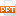# Phân tích dữ liệu bằng phương pháp đánh giá ngẫu nhiên

Data collected from CV survey: HH characteristics Attitude, knowledge, use Program characteristics Design characteristics26 trang | Chia sẻ: aloso | Ngày: 22/08/2013 | Lượt xem: 1494 | Lượt tải: 2
Bạn đang xem nội dung tài liệu Phân tích dữ liệu bằng phương pháp đánh giá ngẫu nhiên, để tải tài liệu về máy bạn click vào nút DOWNLOAD ở trên
Bài giảng 15b: Phân tích dữ liệu CVM Trương Đăng Thụy Sampling techniques Non-Probabilistic Convenient sample: asembles sample at the convenience of researcher Judgement sample: a panel of respondents judged to be representative of the target population is assembled. Quota sample: Selection is controlled by interviewer, ensuring that sample contain given proportion of various types of respondents. Sampling techniques Probabilistic Simple random sampling: every respondents in the sample frame has the same chance of being selected. Systematic sampling: select every kth respondent from a randomly-ordered population frame. Stratified sampling: sampling frame is divided into sub-populations (strata), using random sampling for each stratum. Clustered sampling: population is divided into a set of groups (clusters), and clusters are randomly selected. All elements in the chosen clusters will be included. Multi-stage sampling: random sample of elements within the randomly-chosen clusters. Sample size Coefficient of variation: Necessary sample size: If V=1, =.05 (for Z=1.96), =.1. Then sample size must be 385. In this session Data of WTP Estimating mean and median WTP Non-parametric Parametric Testing validity of WTP values Exercise Data of WTP Three types of CV data: Continuous data (results from open-ended or bidding game questions) Binary data (response “yes” or “no” to a bid level) Interval data (payment card or double-bounded choice) Estimating mean and median WTP: non-parametric Continuous data Imagine a dataset of max WTP of HH/ind Total number of HH is N There are J diferent values of WTP. J might be smaller than N for there could be several HH/ind reporting the same WTP Order the values of WTP Cj from lowest to highest (J=0,J). C0 is always zero and CJ is largest in the sample Let hj is the number of HH/ind in the sample with WTP of Cj Total number of HH/ind with a WTP greater than Cj will be The survivor function is Mean WTP is Estimating mean and median WTP: non-parametric Binary data Total number of respondents is N The sun-sample facing Bj is Nj. The number of respondents saying “Yes” to amount Bj is nj. Survivor function: Mean WTP is Estimating mean and median WTP: non-parametric Binary data – increasing survivor function Calculate Beginning with the first bid level, compare S(Bj) with S(Bj+1) If S(Bj+1) is less than or equal S(Bj), continue If S(Bj+1) > S(Bj), pool the observations of the two bid levels and recalculate the survivor function: Continue until survivor function is non-increasing Mean WTP is Estimating mean and median WTP: non-parametric Interval data: WTP lies in a range lower BL and upper BH Example intervals – non-overlapping Estimating mean and median WTP: non-parametric Interval data: Non-overlapping: use the lower bound and calculate as continuous data Overlapping: may occur in double-bounded dichotomous choice Resp is offered an initial bid If yes, follow up with a higher amount If no, lower amount Estimating mean and median WTP: non-parametric Interval data: WTP will fall in ranges: Yes to B and Yes to BH: WTP lies from BH to ∞ Yes to B and No to BH: WTP lies in the interval of B to BH No to B and Yes to BL: WTP lies in [BL,B] No to B and No to BL: WTP lies in [0,BL] Example intervals and data Estimating mean and median WTP: non-parametric Break overlapping intervals into basic ints Starting from: Using basic intervals only, the probability of lying in basic interval j from Bj-1 to Bj is: Consider overlapping interval of Bi to Bk that spans the basic interval j Estimating mean and median WTP: non-parametric Break overlapping intervals into basic ints Calculate conditional probability of resp whose WTP is in interval Bi to Bk having a WTP that lies in basic interval j: Multiply this probability by number of resp whose WTP is in interval Bi to Bk to obtain estimated number of resp falling in the basic interval j. Estimating mean and median WTP: non-parametric Break overlapping intervals into basic ints Continue the process for all overlapping, we obtain the survivor function for basic intervals only. Then estimate WTP: Total number of HH/ind with a WTP greater than the boundary value Bj will be The survivor function is Mean WTP is Confidence intervals from non-parametric estimation Continuous data Variance of population WTP Estimate of variance of mean WTP Confidence interval (95%) Confidence intervals from non-parametric estimation Binary data Estimate of variance of mean WTP Confidence interval (95%) Parametric estimation of mean and median WTP The mean and meadian WTP is: Restricted mean/median WTP: Testing validity of WTP values Test whether WTP values provided follow distinguishable patterns, conforming prior expectations and economic theory Regress WTP on a numbers of variables: Income Socio-economic characteristics Attitudinal variables Attitude toward CV program design Knowledge on the good provided Proximity to the site of provision Testing validity of WTP values To test: Regress WTP on variables Test for significance of coefficients (t-test can be used) Examine the sign of coefficients. Are they consistent with economic theory? Look at pseudo-R2. Should not be less than 0.1. Exercise Use the provided data sets (binary and interval data) to calculate: Mean/Median WTP 95% confidence interval for each data set Exercise: Binary data Exercise: Interval data Data of WTP Data collected from CV survey: HH characteristics Attitude, knowledge, use Program characteristics Design characteristics Data of WTP Missing data on WTP: Refuse to answer the valuation questions Provide zero value instead of true value Provide unrealistically high value On HH characteristics: impute values

Các file đính kèm theo tài liệu này:

•Bài giảng 15b - Phân tích dữ liệu CVM.ppt
Tài liệu liên quan